Structural Bioinformatics Library Template C++ / Python API for developping structural bioinformatics applications.
User Manual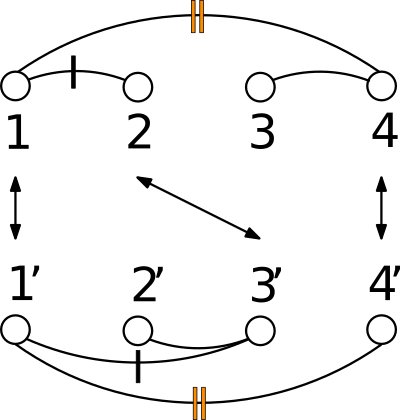# Apurva

Authors: N. Malod-Dognin and R. Andonov

# Introduction

## CMO: Contact Map Overlap

Given a polypeptide chain, a protein contact map is a binary matrix such that the i-j-th element is one if the two corresponding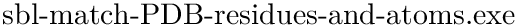are within a user-defined distance threshold, and zero otherwise. Alternatively, a contact map can be represented as a graph, with an edge between twoiff the corresponding entry of the matrix is one.

In the CMO approach, the similarity between two proteins is determined by the extent of overlap of their contact maps.

See Protein Contact Map for more detail, and Fig. fig-CMO for an illustration.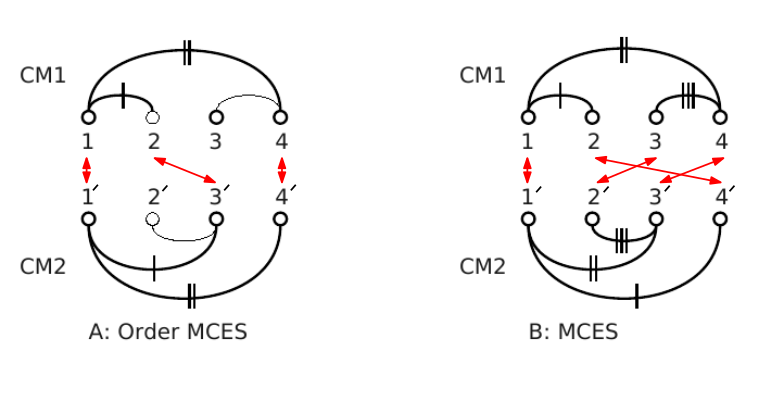Contact Map Overlap (A)Vertex set (1, 2, 4) from CM1 is matched with vertex set (1', 3', 4') from CM2. Common edges (two in this case) are denoted by the same number of vertical dashes. This is an order preserving MCES with maximum score 2. (B) Vertex set (1, 2, 3, 4) from CM1 is matched with vertex set (1', 4' , 2', 3') from CM2. This is a MCES with score 3. The order is not preserved.

The formal definition of CMO is as follows. Let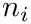and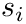be arbitrary subsets of vertices from the first and second contact maps, respectively.

Under the alignment (matching, one-to-one map)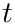, the edge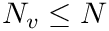is common (an overlap occurs) if and only if both edges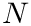and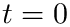exist in the two contact maps processed.

The CMO problem is to find the optimal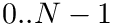and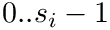, where optimality means maximum number of common edges. Since the sets of amino-acids are supposed linearly ordered, crossings are not allowed (see Fig. fig-CMO).

Apurva is a Contact Map Overlap maximization (CMO) solver. Given two protein structures represented by two contact maps, Apurva computes the amino-acid alignment that maximizes the number of common contacts. More information about the solver can be found in the articles  and  .

## Format for contact maps

Apurva can process contact maps. A contact map file is divided in three sections, separated by an empty line.

• The first section describes the contacts between the amino-acids. The first line contains an integer value X, which is the number of residues. Note that residues are labelled starting from one. The second line contains an integer value Y, which is the number of contacts. The next Y lines each contain two integer values i and j, i<j, the tail and head residues of each contact.

• The second section describes the secondary structure assignment of the protein. It start with a line containing an integer value S, which is the number of secondary structure elements (SSE). It is then followed by S lines, each containing two integers (i and j) and a symbol t, where i is the first amino-acid of the SSE, j is the last amino-acid of the SSE, and t is the type of the SSE (either H for an alpha-helix, or b for a beta-strand).

• The last section describes the contacts between the secondary structure elements. It starts with a line containing an integer value C, which is the number of contacts between the SSE. It is then followed by C lines, each containing three integer values i, j and k, corresponding to the contact between SSE i and the SSE j, i<j, and having a weight k, which is the number of amino-acid contacts between the two SSEs.

The contact map files in the sequel were generated as follows: parameters:

• Contact distance threshold between alpha carbons is 7.5A.
• Contacts between consecutive amino-acids (i and i+1) are not taken into account.
• Secondary structure assignments, computed e.g. using KAKSI  .

Here is the contact map file for chain A in file 1vfb.pdb :

107
372
0 2
...
104 106

10
3 6 b
...
101 105 b
13
0 2 13
...
8 9 9


The first line indicates the number of residues, the second line the number of contacts, and then all contacts are enumerated by residue identifiers. After a blank line, the number of secondary structure elements (SSE) are indicated. Then, the start residue, the end residue, and the type of SSE (b for beta-sheet and H for alpha-helix). Finally, the number of contacts between SSE is followed by the SSE identifiers and the number of residues in contact between the two SSE.

# Algorithm

CMO is an NP-hard problem  . The algorithms used here to solve CMO provided here are presented in  and  . These algorithms use an an integer programming model for CMO, and solve it with an an exact branch-and-bound algorithm with bounds obtained by a novel Lagrangian relaxation.

# Examples

## Executable: sbl-apurva.exe

In this section, we present the executable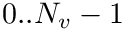, provided with the SBL .

Restrictions on PDB files.

Apurva can process .PDB files (.pdb or .ent), with the following restrictions:

• (1) One chain per PDB file
• (2) The PDB files should not contain more than one model
• (3) The HETATM are not processed

Options.

The main options of the program(PDB file specific options) are:
–pdb1 string: First .pdb file (used as row)
–pdb2 string: Second .pdb file (used as col)
–chain1 string: Id of the chain that will be processed in the first pdb file
–chain2 string: Id of the chain that will be processed in the second pdb file
–dthr float(=7.5): Distance threshold for generating contacts, in angstrom
–vmd bool(=0): If set to 1, generate visualisation file for VMD

The main options of the program(Contact map specific options) are:
–cm1 string: First contact map file (used as row)
–cm2 string: Second contact map file (used as col)

The main options of the program(General options) are:
–solventfilter bool(=0): Amino-acid alignment filter based on solvent accessibility, as derived from contact density (0->Do not use solvent accessibility filter,1->Use solvent accessibility filter)
–ssefilter int(=0): Amino-acid alignment filters based on secondary structure elements (SSE) (0->No SSE filter, 1->Matchings between alpha helices and beta-stands are prohibited, 2->Also prohibits matchings between secondary structure elements and loops)
–hierarchical bool(=0): Amino-acid alignment filters based on secondary structure elements (SSE) (0->No SSE filter, Hierarchical approach: first align the secondary structure elements, and second extend the alignment to the amino (0->Do not use the hierarchical approach), 1->Use the hierarchical approach)
–sseonly bool(=0): If set to 1, Apurva only computes secondary structure alignment
–display int(=0): Set the display level of Apurva (0-> Display only the comparison scores, 1-> Comparison scores + statistics, 2 -> Comparison score + statistics + alignment)
–nodelimit int(=10000000): Maximum number of branch and bound nodes to be explored (0 -> root node only)
–iterlimit int(=4000): Maximum number of iteration per Branch and Bound node
–timelimit float(=1800): Maximum execution time (in seconds). Note that the time limit is checked after each iteration, and thus it is not very accurate.

Examples.

Apurva will align the two contact maps 1amkA.hcm and 1aw2A.hcm, and will display the corresponding alignment :

./sbl-apurva.exe --cm1 ./1amkA.hcm --cm2 ./1aw2A.hcm --display 2


Apurva will align chain A of 1amk.pdb with chain A of 1aw2.pdb, and will generate two files for VMD that highlight the matching between the two protein chains :

./sbl-apurva.exe --pdb1 1amk.pdb --chain1 A --pdb2 1aw2.pdb --chain2 A --vmd 1